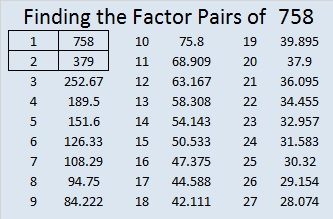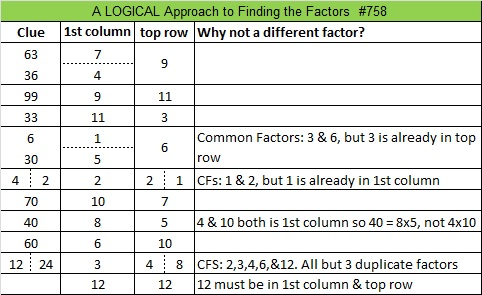# 758 and Level 5

• 758 is a composite number.
• Prime factorization: 758 = 2 x 379
• The exponents in the prime factorization are 1 and 1. Adding one to each and multiplying we get (1 + 1)(1 + 1) = 2 x 2 = 4. Therefore 758 has exactly 4 factors.
• Factors of 758: 1, 2, 379, 758
• Factor pairs: 758 = 1 x 758 or 2 x 379
• 758 has no square factors that allow its square root to be simplified. √758 ≈ 27.5317998.I started to feel like I was getting behind blogging so I decided over the weekend that it is okay NOT to blog everyday. I didn’t have time to post this puzzle until today, and I have a level 6 puzzle and another whole set of puzzles to post as well. I decided I don’t need to catch up. I’ll post them all soon enough. A logical way to find the solution is in a table at the end of the post.Print the puzzles or type the solution on this excel file: 12 Factors 2016-01-25

—————————————

Here’s more about the number 758:

758 is the sum of four consecutive numbers: 188 + 189 + 190 + 191 = 758.

758 is the sum of three squares six different ways:

• 27² + 5² + 2² = 758
• 26² + 9² + 1² = 756
• 23² + 15² + 2² = 758
• 22² + 15² + 7² = 758
• 21² + 14² + 11² = 758
• 19² + 19² + 6² = 758

758 is a palindrome in two different bases:

• 464 BASE 13; note that 4(13²) + 6(13) + 4(1) = 758.
• 262 BASE 18; note that 2(18²) + 6(18) + 2(1) = 758.

—————————————This site uses Akismet to reduce spam. Learn how your comment data is processed.